## RS Aggarwal Class 9 Solutions Chapter 8 Linear Equations in Two Variables Ex 8A

These Solutions are part of RS Aggarwal Solutions Class 9. Here we have given RS Aggarwal Class 9 Solutions Chapter 8 Linear Equations in Two Variables Ex 8A.

Question 1.
Solution:
(i) x = 5 is the line AB parallel to the y-axis at a distance of 5 units.
(ii) y = – 2 is the line CD parallel to x-axis at a distance of – 2 units.
(iii) x + 6 = 0 => x = – 6 is the line EF parallel to y-axis at a distance of – 6 units.
(iv) x + 7 = 0 => x = – 7 is the line PQ parallel to y-axis at a distance of – 7 units.
(v) y = 0 is the equation of x-axis. The graph of y = 0 is the line X’OX
(vi) x = 0 is the equation of y-axis.The graph of x = 0 is the line YOY’
Ans.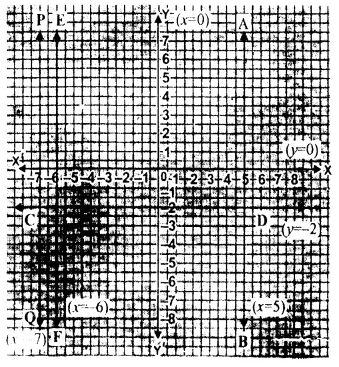Question 2.
Solution:
In the given equation.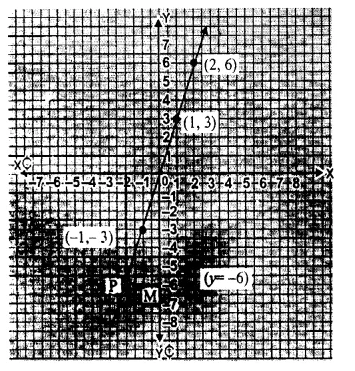y = 3x
Put x = 1, then y = 3 x 1 = 3
Put x = 2, then y = 3 x 2 = 6
Put x = – 1, then y = 3 ( – 1) = – 3
Now, plot the points (1, 3), (2, 6) and ( – 1, – 3) as given the following table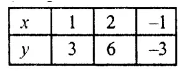and join them to form a line of the given equation.
Now from x = – 2,
draw a line parallel to y-axis at a distance of x = – 2, meeting the given line at P. From P, draw, a line parallel to x-axis joining y-axis at M, which is y = – 6 Hence, y = – 6 Ans.

Question 3.
Solution:
In the given equation x + 2y – 3 = 0
=> 2y = 3 – x
y = $$\frac { 3-x }{ 2 }$$
put x = 1,then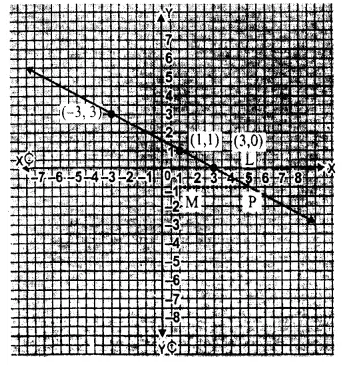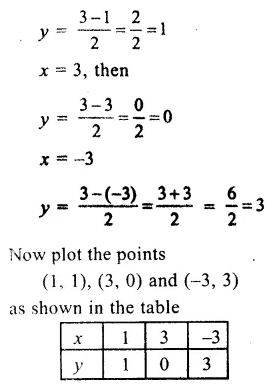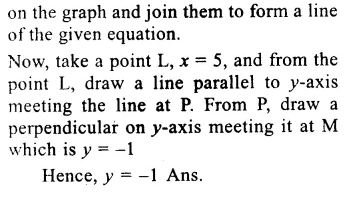Question 4.
Solution:
(i) In the equation y = x
When x = 1, then y = 1
when x = 2, then y = 2
and when x = 3, then y = 3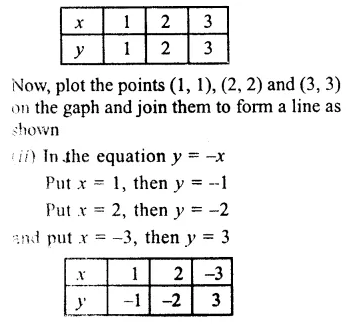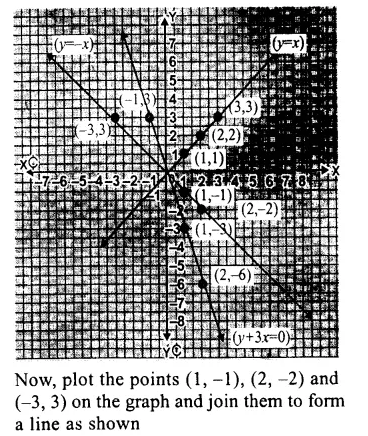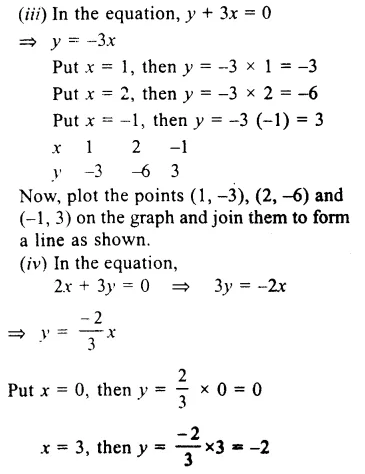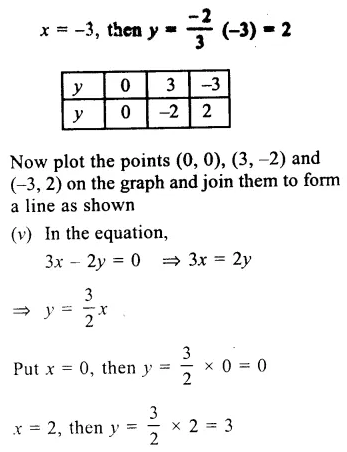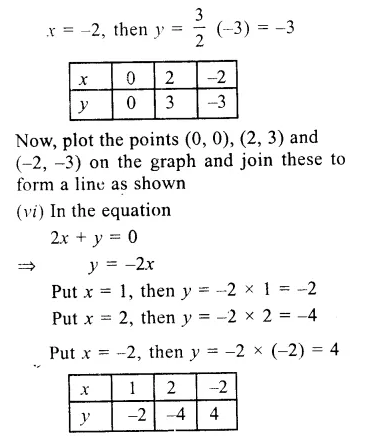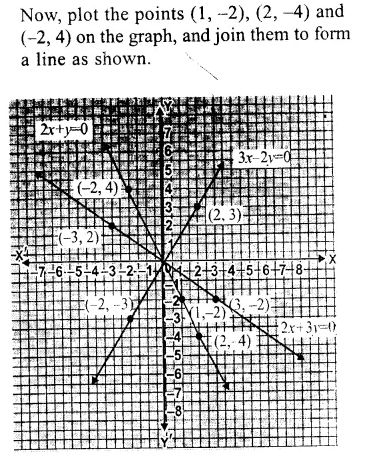Question 5.
Solution:
In the given equation
2x – 3y = 5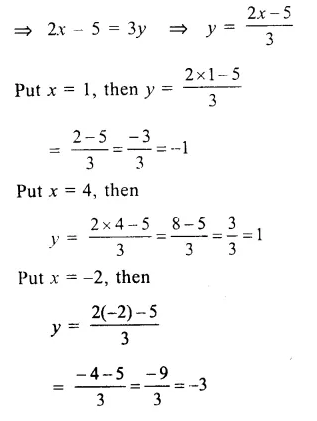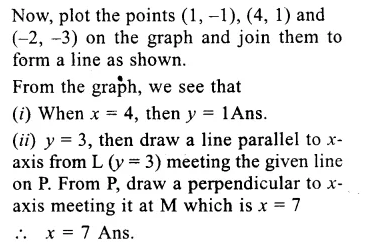Question 6.
Solution:
In the given equation
2x + y = 6
=> y = 6 – 2x
Put x = 1, then y – 6 – 2 x 1 = 6 – 2 = 4
Put x = 2, then y = 6 – 2 x 2 = 6 – 4 = 2
Put x = 3, then y = 6 – 2 x 3 = 6 – 6 = 0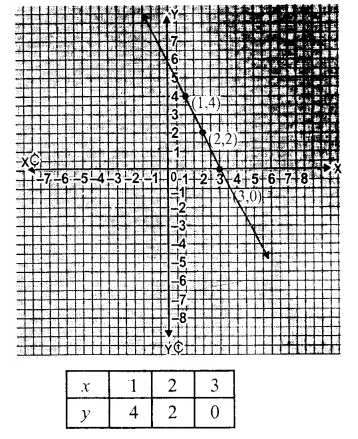Question 7.
Solution:
In the given equation
3x + 2y = 6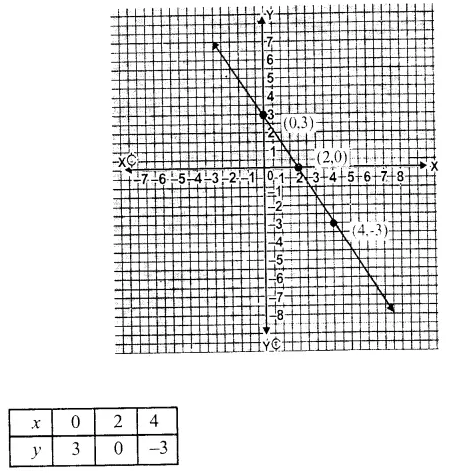Hope given RS Aggarwal Class 9 Solutions Chapter 8 Linear Equations in Two Variables Ex 8A are helpful to complete your math homework.

If you have any doubts, please comment below. Learn Insta try to provide online math tutoring for you.